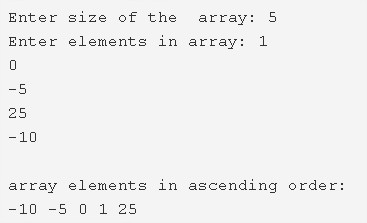# C Program To Sort Array Elements In Ascending Order | 4 Ways

C program to sort the array elements in ascending order – In this article, we will detail in on the aggregation of ways to sort the array elements in ascending order in C programming.

Suitable examples and sample programs have also been added so that you can understand the whole thing very clearly. The compiler has also been added with which you can execute it yourself.

The ways represented in this appropriate piece are as follows:

• Using Standard Method
• Using Function

As we all know, an array is a sequence of a bunch of elements in any given order whatsoever. Arrays are used to display information in that specific order only.As you can see in the image uploaded, the size of the array is entered first up.

The size of the array given in this case is 5.

Eventually, the elements in the array are listed.

The elements in this particular array are 1, 0, -5, 25, -10.

Hence, the descending order of the elements entered is -10, -5, 0, 1, 25.

Thus, the numerous ways to set a bunch of elements of an array in ascending order are as follows:

## Using Standard Method

1. Read the size of the array and store the value into the variable n.

2) Read the entered elements one by one and store the elements in the array a[] using scanf(“%d’&a[i]) and the for loop for(i=0;i<n;i++).

3) Arrange the array elements from least to highest value as

for loop iterates from i=0 to i<n-1

a) Compare a[j] element with adjacent element a[j+1] and find the highest element then exchange the both elements positions using for loop for (j=0;j<n-i-1;j++).

4) After all iterations of for loop, we will get sorted array in which the elements are in ascending order. Print the array elements using the for loop and printf statement.

Output:

### Using Function

1. The main() calls the sort() to sort the array elements in ascending order by passing array a[], array size as arguments.

2) The sort() function compare the  a[j] and a[j+1] with the condition a[j]>a[j+1],if a[j] is the highest value than a[j+1] then swap the both elements.

3)To sort the array in ascending order

a) Repeat the step b  from i=0 to i<n-1

b) for loop iterates from j=0 to j<n-i-1

find the highest element by comparing a[j] and a[j+1] and swap both elements. Repeat until all iterations of j.

4) After all iterations of i, the sorted array will be generated in which the elements are in ascending order.

5) To print the sorted array, the main() function calls the print() function by passing the array, size of the array as arguments.

Output:
x

## C Program To Print Number Of Days In A Month | Java Tutoring

C program to input the month number and print the number of days in that ...